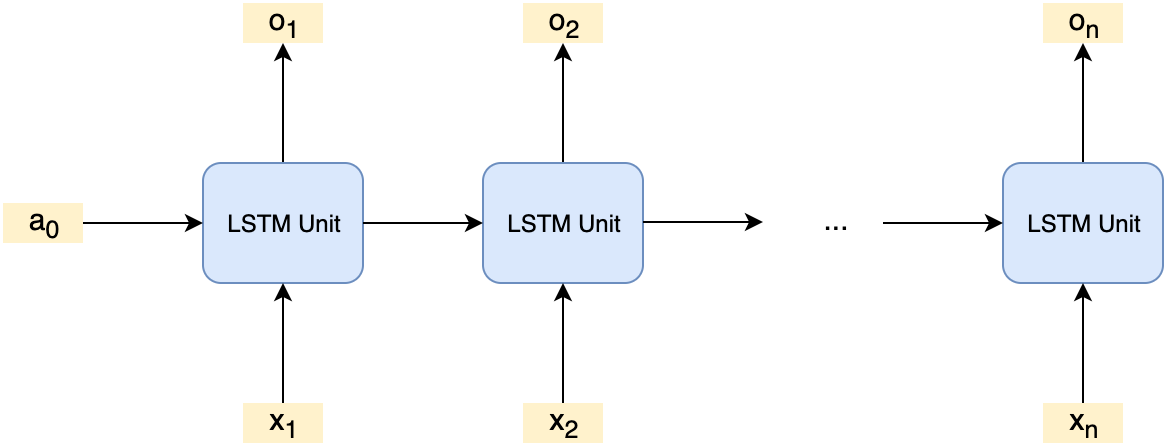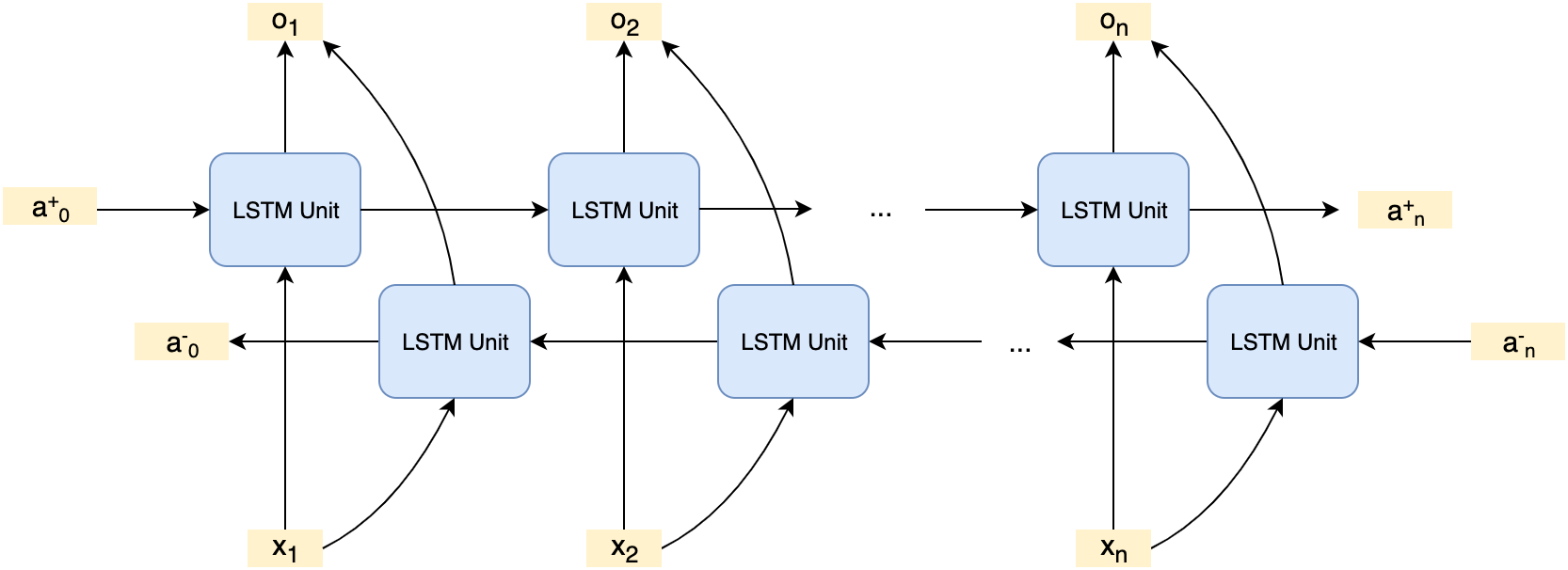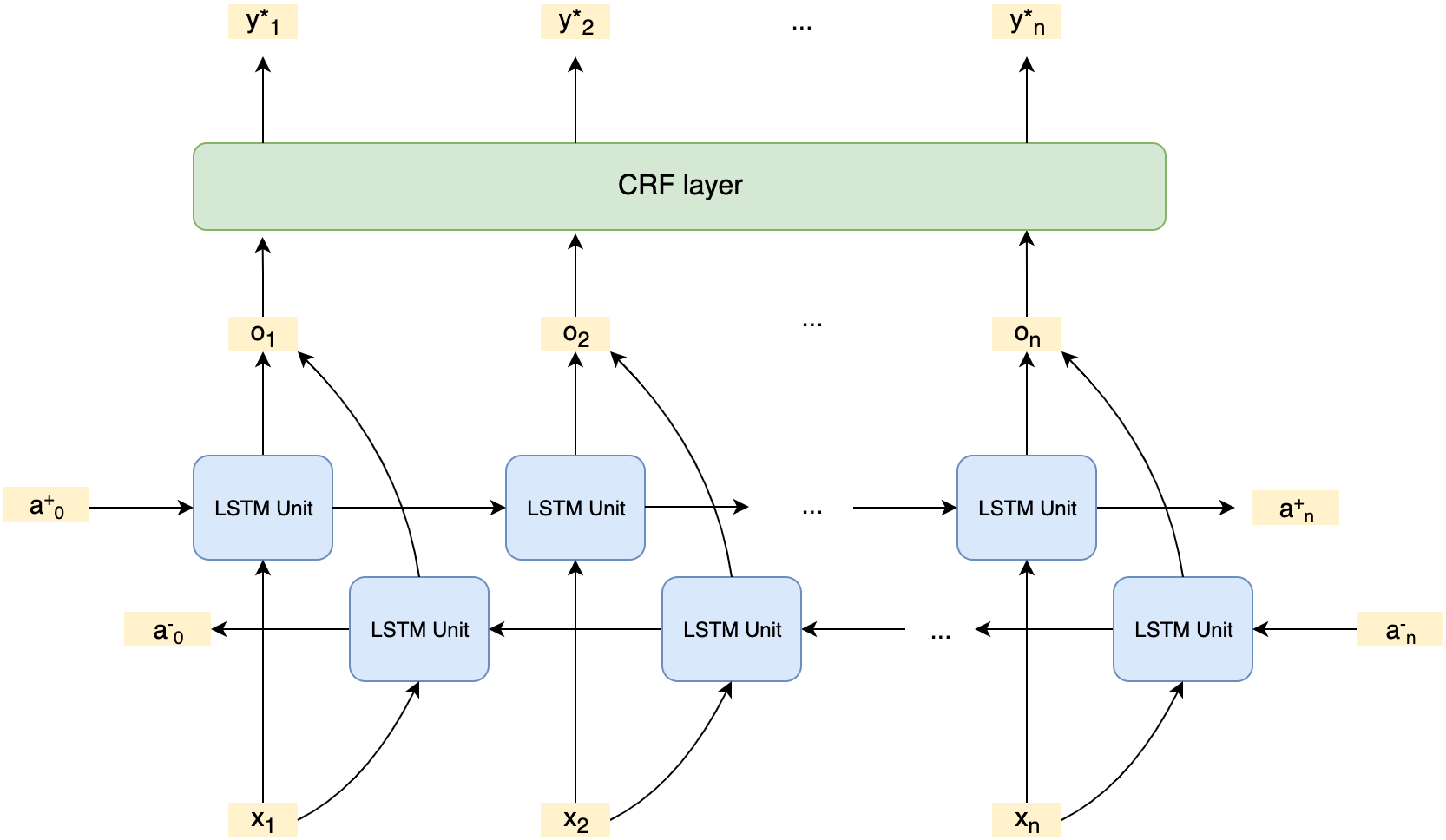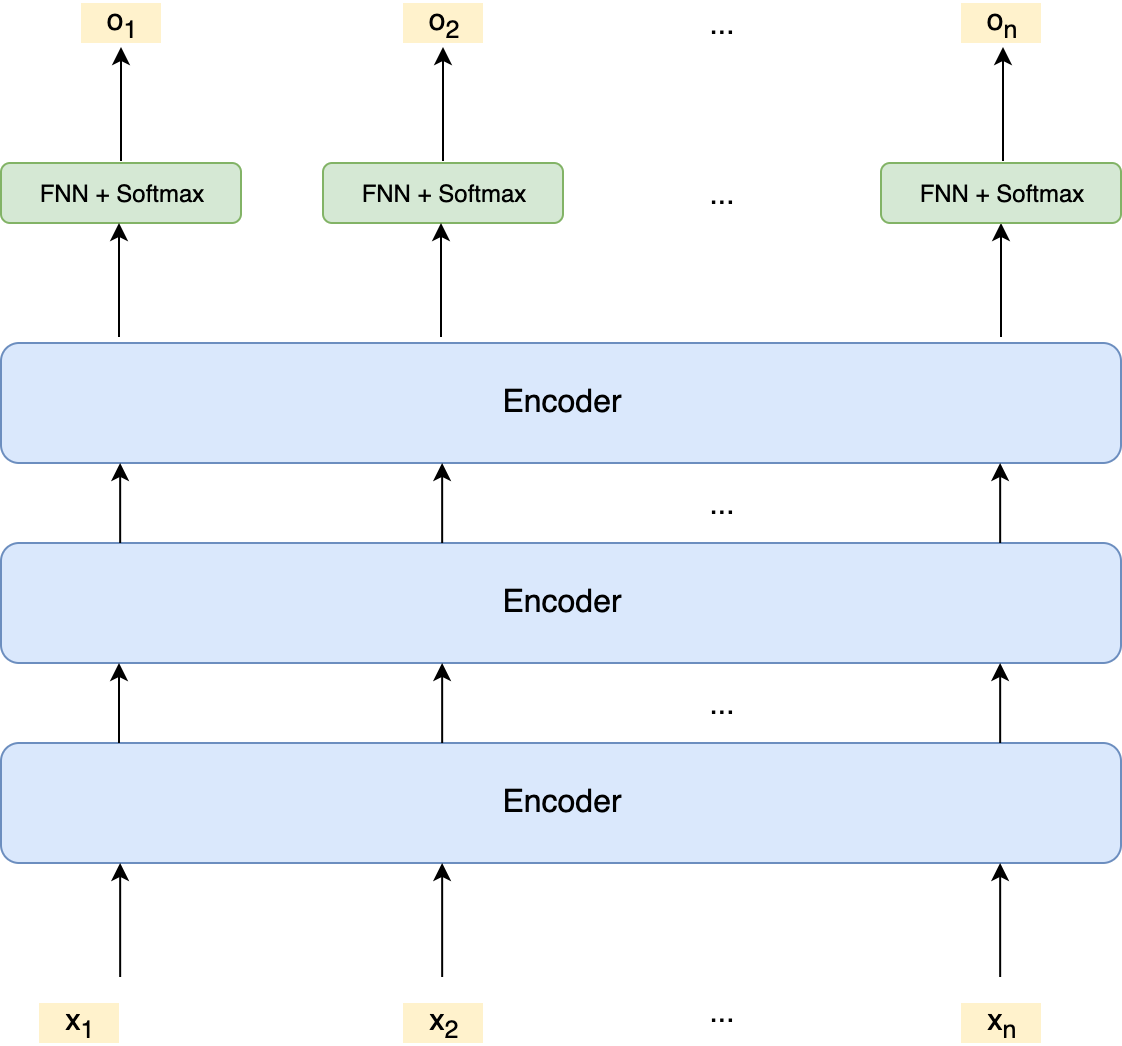As a continuation for Demystifying Named Entity Recognition - Part I, in this post I’ll discuss popular models available in the field and try to cover:

• deep learning models

• python libraries

Over the history of NER, there’s been three major approaches: grammar-based, dictionary-based and machine-learning-based. Grammar-based approach produces a set of empirical rules hand-crafted by experienced computational linguists, usually takes months of work. Dictionary-based approach basically organizes all the known entities into a lookup table, which can be used to detect whether a candidate belongs to a defined category or not. By design it doesn’t work well with newly invented entities. Machine-learning-based approach typically needs annotated data, but doesn’t necessarily rely on domain experts to come up with rules or fail on unseen entities.

This post focuses only on machine-learning based models.

The traditional models we’ll discuss here are MEMM, CRF. They are very popularly used before deep learning models entered the scene.

### 1.1 MEMM

We’ve covered the details of MEMM in the previous post. The key idea of the MEME approach is to model the conditional probability of tag sequenece for a given sentence with Markov assumption:

We then model $p(y_i | y_{i-1}, x_1...x_n)$ using local environment:

In inference, we use Viterbi algorithm to get best-fitting tag sequence for a given sentence. Details can be found in 2.2.1 section of the previous post.

In training, we use maximum likelihood estimation to get optimal $\underline{\theta}$ that

where $\underline{x}^j, \underline{y}^j$ are the $j^{th}$ sentence and corresponding tag sequence (the whole training dataset has $N$ examples).

### 1.2 CRF

Instead of $p(y_i | y_{i-1}, \underline{x})$ , Conditional Random Field (CRF) approach chooses to directly model $p(\underline{y} | \underline{x})$:

The main challenge in direct modeling is that the denominator is sum of $K^n$ terms where $K$ is the number of tag label types and $n$ is the length of sentence to tag. This is a much larger number than that in MEMM - $p(y_i | y_{i-1}, x_1...x_n)$ has just $K$ terms in the denominator.

#### 1.2.1 Inference

During inference, we are only interested in the $\underline{y}^{*}$ that gives the highest probability rather than the highest probability itself:

If using brutal force, we have to evaluate $\exp({\underline{\Theta} \cdot \underline{F}(\underline{x}, \underline{y})})$ for $K^n$ times.

Fortunately, if we add a little structure into $\underline{F}(\underline{x}, \underline{y})$, which I’m going to talk about next, we can bring the exponential complexity - $O(K^n)$ down to linear complexity - $O(K^2n)$.

The structure added in CRF is:

To maximize $\underline{\Theta} \cdot \underline{F}(\underline{x}, \underline{y})$, we define a partial score as we did in 2.2.1 section of the previous post:

If we can maximize any partial score (which turns out not that difficult), then the score we want to acutally maximize, $\underline{\Theta} \cdot \underline{F}(\underline{x}, \underline{y})$, is just a special case of $s_{partial, k}$ when $k=n$.

So how to maximize any partial score? Let’s start with $k=1$, namely $s_{partial, 1} (y_1)= \underline{\Theta} \cdot \underline{f}(\underline{x}, y_1)$.

This is easy because it’s just a single-variable optimization and $y_1$ can only have K choices. We also store all the evaluated $s_{partial, 1} (y_1)$.

How about $k=2$, namely maximize $s_{partial, 2}(y_1, y_2) = s_{partial, 1}(y_1) + \underline{\Theta} \cdot \underline{f}(\underline{x}, y_1, y_2)$?

We can first fix $y_2$ and optimize over $y_1$ dimension. Remember we’ve known $s_{partial, 1}(y_1)$ evaluated from the previous question. So it takes $K$ computations to find the optimal $y_1$ for each $y_2$ - $s_{partial, 2}(y_1^*, y_2)$. Then pick the $y_2^*$ which has maximum $s_{partial, 2}(y_1^*, y_2)$. In total, we need perform $K^2$ evaluations. We also store all the $s_{partial, 2}(y_1^*, y_2)$ for future use.

How about $k=3$, namely maximize $s_{partial, 3}(y_1, y_2, y_3) = s_{partial, 2}(y_1, y_2) + \underline{\Theta} \cdot \underline{f}(\underline{x}, y_2, y_3)$?

Similar to the previous question, we try to estimate $s_{partial, 3}(y_1^*, y_2^*, y_3)$ for each $y_3$ using $s_{partial, 3}(y_1^*, y_2^*, y_3) = \max_{y_2}(s_{partial, 2}(y_1^*, y_2) + \underline{\Theta} \cdot \underline{f}(\underline{x}, y_2, y_3))$. We also carry out $K$ evaluation per $y_3$, thus totally $K^2$ evaluations for all possible $y_3$. We store $s_{partial, 3}(y_1^*, y_2^*, y_3)$ for future use (e.g., when $k=4$).

By doing this all the way to $k=n$, we can get $\max_{\underline{y}}{\underline{\Theta} \cdot \underline{F}(\underline{x}, \underline{y})}$ with roughly $K^2n$ evaluations.

#### 1.2.2 Training

Similar to MEMM, we can also use maximum likelihood estimation to get optimal $\underline{\Theta}$ that

where $\underline{x}^j, \underline{y}^j$ are the $j^{th}$ sentence and corresponding tag sequence (the whole training dataset has $N$ examples). More details on training algorithm can be found in Page 10 of Michael Collins’s CRF note.

## 2. Deep learning models

The deep learning models we’ll discuss here are LSTM, BiLSTM-CRF, Bert.

### 2.1 LSTM

#### 2.1.1 ArchitectureIn the setting of LSTM, each token $x_i$ is fed to a LSTM unit, which outputs a $o_i$. $o_i$ models log probabilities of all possible tags at i-th position, so it has dimension of $K$.

#### 2.1.2 Inference

The inference in LSTM is very simple: $y_i$ = the tag with highest log probability at i-th position.

which indicates the prediction of i-th position only utilizes the sentence information up to i-th token - only the left side of the sentence is used for tag prediction at i-th position. BiLSTM is designed to provide context information from both sides, which will be seen in next section.

#### 2.1.3 Training

Like all the other neural network training, LSTM training uses Stochastic Gradient Descent algorithm. Loss function adopts negative log likelihood. For a data point $(\underline{x^j}, \underline{y^j})$, we have its loss calculated as:

where $n_j$ is the length of the sentence $x^j$, $o_i^j$ is the LSTM output at i-th position and $y_i^j$ is the ground truth tag at i-th position.

Total loss is the mean of all the individual losses.

where $N$ is the total number of training examples.

### 2.2 BiLSTMBiLSTM stands for bi-directional LSTM, which provides sequence information from both directions. Because of that, BiLSTM is more powerful than LSTM. Except the bi-directional component, the meaning of network output, inference, and training loss are same as LSTM.

### 2.3 BiLSTM-CRF

BiLSTM captures contextual information around i-th position. But at each position, BiLSTM predicts tags basically in an independent fashion. There’s cases where some adjacent positions are predicted with tags which do not usually appear together in reality. For example, I-PER tag should not follow B-ORG. To account for this kind of interactions between adjacent tags, Conditional Random Field (CRF) is introduced to BiLSTM.

#### 2.3.1 Architecturewhere $o_i$ models emission scores of all possible tags at i-th position and $y_i^*$ is the best tag for i-th position which collectively achieves highest sequence score.

CRF layer also learns a transition matrix $A$ which stores transition scores between any possible pair of tag types.

#### 2.3.1 Inference

Same as the inference in CRF section, given a trained network and sentence $\underline{x}$, any sequence $\underline{s}$ will have a score.

The score is a sum of contributions from token level. i-th position has contribution of $\phi(\underline{x}, s_{i-1}, s_i) = o_i[s_i] + A[s_{i-1}][s_i]$, where the first term is emission score and second term is transition score.

To find the tag sequence $\underline{y}^*$ achieving highest score, we need to use dynamic programming.

Define sub problem $DP(k,t)$ to be the max score accumulated from 1st position to $k$-th position with the $k$-th position tag being $t$, detailed as follows:

The recursion would be:

The original problem is then

We can always use parent pointers to retrieve the corresponding best sequence $\underline{y}^*$.

#### 2.3.2 Training

Loss function for BiLSTM-CRF also adopts negative log likelihood. For a data point $(\underline{x^j}, \underline{y^j})$, we have its loss calculated as:

where the first term is easy to calculate via a forward pass of the network and the second term needs more care. Let’s define that term (without log) as $Z$, which is exponential sum of scores of all the possible sequences $\underline{s}$ of length $n$.

To calculate $Z$, we need to use dynamic programming again. This time the sub-problem $DP(k,t)$ is the exponential sum of scores of all possible sequences of length $k$ with last tag $s_k = t$:

The recursion would be:

The original problem is then

Via this way, individual loss $L_j$ is calculated and then batch loss by averaging the individual losses in the batch.

### 2.4 Bert

Recent research on BERT provides an option for NER modeling. Despite of the complexity of the BERT model architecture, in the context of NER it can be regarded as an advanced version of our BiLSTM model - replacing the LSTM with multiple Transformer Encoder layers.Thus, $o_i$ still models log probabilities of all possible tags at i-th position.

Inference, and training loss are same as LSTM section.

## 3. Python libraries

There’s several machine learning based NER repositories in GitHub. I picked some of them here with some comments.

• KEHANG/ner: for English texts, based on PyTorch, has LSTM, BiLSTM, BiLSTM+CRF and Bert models, has released conda package

• shiyybua/NER: for Chinese texts, based on Tensorflow, only BiLSTM+CRF model, no packages released

• Franck-Dernoncourt/NeuroNER: for English texts, based on Tensorflow, has LSTM model, no package released